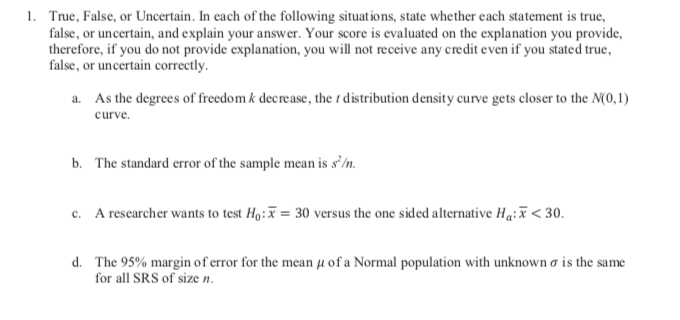# True, False, or Uncertain. In each of the following situations, state whether each statement is true,false, or uncertain, and explain your answer. Your score is evaluated on the explanation you providetherefore, if you do not provide explanation, you will not receive any credit even if you stated truefalse, or uncertain correctly1.As the degrees of freedom k decrease, the t distribution density curve gets closer to the N(0,1)curvea.The standard error of the sample mean is s'/nb.A researcher wants to test Ho:= 30 versus the one sided alternative Ha:< 30c.d.The 95% margin of error for the mean u of a Normal population with unknown o is the samefor all SRS of size n.

Question
211 viewshelp_outlineImage TranscriptioncloseTrue, False, or Uncertain. In each of the following situations, state whether each statement is true, false, or uncertain, and explain your answer. Your score is evaluated on the explanation you provide therefore, if you do not provide explanation, you will not receive any credit even if you stated true false, or uncertain correctly 1. As the degrees of freedom k decrease, the t distribution density curve gets closer to the N(0,1) curve a. The standard error of the sample mean is s'/n b. A researcher wants to test Ho:= 30 versus the one sided alternative Ha:< 30 c. d. The 95% margin of error for the mean u of a Normal population with unknown o is the same for all SRS of size n. fullscreen
check_circle

star
star
star
star
star
1 Rating
Step 1

Note:

Thank you for the question. As your question has more than 3 parts, according to our policy, we are answering the first 3 parts for you. If you need help with any other particular part, please re-post the question and mention the part you need help with.

Step 2

a. False,

As the degrees of freedom increases, the t-distribution approaches normality. This means that, the area near the center increases while the area near the tail decreases.  When the degrees of freedom decreases, the area near the center decreases while the area near the tail increases. Or otherwise, as the degrees of freedom increases, the sample size increases and thus, the sample approaches the population.

Thus, the statement that ‘As the degrees of freedom k decrease, the t distribution density curve gets closer to the N(0,1) curve’ is False.

Step 3

b. False,

The standard error of the mean obtained by dividing the sample standard deviation with the square root of sample size. That is, SE= s/sqrt(n).

...

### Want to see the full answer?

See Solution

#### Want to see this answer and more?

Solutions are written by subject experts who are available 24/7. Questions are typically answered within 1 hour.*

See Solution
*Response times may vary by subject and question.
Tagged in

### Other